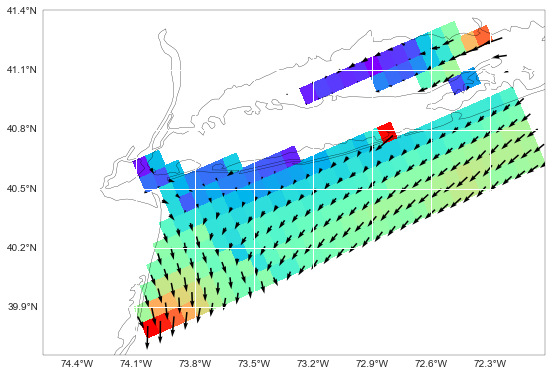# Python tools for sgrid

The pysgrid module implements the sgrid conventions akin to pyugrid and the UGRID conventions.

I will not get into the details of the convention. This post is just a copy of the pysgrid notebook example with a few comments to help people use it.

Note that, even thought many ocean models out there using staggered grids, very few are SGRID compliant. The convention is very new and the tools to bring model results up to compliance are still being developed.

The URL below, for example, was modified via an ncml aggregation to comply with the SGRID standards.

In :
from netCDF4 import Dataset

url = ('http://geoport.whoi.edu/thredds/dodsC/clay/usgs/users/'
'jcwarner/Projects/Sandy/triple_nest/00_dir_NYB05.ncml')

nc = Dataset(url)


The creation of the object is a little bit slow. There is some room for improvement like deferring some of the loading/computations.

In :
import pysgrid

%time sgrid = pysgrid.from_nc_dataset(nc)

CPU times: user 39 ms, sys: 7 ms, total: 46 ms
Wall time: 34.4 s



The object knows about sgrid conventions.

In :
sgrid.edge1_coordinates, sgrid.edge1_dimensions, sgrid.edge1_padding

Out:
((u'lon_u', u'lat_u'),
u'xi_u: xi_psi eta_u: eta_psi (padding: both)',

In :
u_var = sgrid.u
u_var.center_axis, u_var.node_axis

Out:
(1, 0)

In :
v_var = sgrid.v
v_var.center_axis, v_var.node_axis

Out:
(0, 1)


And builds the slicers to get the padded variables ready to be averaged at the center of the grid.

In :
u_var.center_slicing

Out:
(slice(None, None, None),
slice(None, None, None),
slice(1, -1, None),
slice(None, None, None))

In :
v_var.center_slicing

Out:
(slice(None, None, None),
slice(None, None, None),
slice(None, None, None),
slice(1, -1, None))


The API is still a little bit rough. To perform a centered grid average we need to:

1) get the variables

In :
u_velocity = nc.variables[u_var.variable]
v_velocity = nc.variables[v_var.variable]


2) apply the center_slicing to each variable

In :
from datetime import datetime, timedelta
from netCDF4 import date2index

t_var = nc.variables['ocean_time']
start = datetime(2012, 10, 30, 0, 0)
time_idx = date2index(start, t_var, select='nearest')

v_idx = 0

# Slice of the slice!
u_data = u_velocity[time_idx, v_idx, u_var.center_slicing[-2], u_var.center_slicing[-1]]
v_data = v_velocity[time_idx, v_idx, v_var.center_slicing[-2], v_var.center_slicing[-1]]


3) average the sliced variables

In :
from pysgrid.processing_2d import avg_to_cell_center

u_avg = avg_to_cell_center(u_data, u_var.center_axis)
v_avg = avg_to_cell_center(v_data, v_var.center_axis)


The processing_2d submodule brings some extra (non-SGRID) functionality that is very useful. Like rotation and vector sum.

In :
from pysgrid.processing_2d import rotate_vectors

angles = nc.variables[sgrid.angle.variable][sgrid.angle.center_slicing]
u_rot, v_rot = rotate_vectors(u_avg, v_avg, angles)

In :
from pysgrid.processing_2d import vector_sum

uv_vector_sum = vector_sum(u_rot, v_rot)


The pysgrid module inherited some of the pyugrid quirks, like packing the coordinates into one array. That is awkward for 2D coordinates.

Here is how to get the grid centers via the raw pysgrid API.

In :
grid_cell_centers = sgrid.centers

lon_var_name, lat_var_name = sgrid.face_coordinates

sg_lon = getattr(sgrid, lon_var_name)
sg_lat = getattr(sgrid, lat_var_name)

lon_data = grid_cell_centers[..., 0][sg_lon.center_slicing]
lat_data = grid_cell_centers[..., 1][sg_lat.center_slicing]


Now that we have data and coordinates at the grid center we can finally plot the data!

In :
import numpy as np
import matplotlib.pyplot as plt

import cartopy.crs as ccrs
from cartopy.mpl.gridliner import LONGITUDE_FORMATTER, LATITUDE_FORMATTER

def make_map(projection=ccrs.PlateCarree(), figsize=(9, 9)):
fig, ax = plt.subplots(figsize=figsize,
subplot_kw=dict(projection=projection))
gl = ax.gridlines(draw_labels=True)
gl.xlabels_top = gl.ylabels_right = False
gl.xformatter = LONGITUDE_FORMATTER
gl.yformatter = LATITUDE_FORMATTER
return fig, ax

In :
sub = 10
scale = 0.06

fig, ax = make_map()

kw = dict(scale=1.0/scale, pivot='middle', width=0.003, color='black')
q = plt.quiver(lon_data[::sub, ::sub], lat_data[::sub, ::sub],
u_rot[::sub, ::sub], v_rot[::sub, ::sub], zorder=2, **kw)

cs = plt.pcolormesh(lon_data[::sub, ::sub],
lat_data[::sub, ::sub],
uv_vector_sum[::sub, ::sub], zorder=1, cmap=plt.cm.rainbow)

_ = ax.coastlines('10m')Those familiar with a specific model tool, like pyroms and octant, might dismiss this example and keep using a more convenient way to perform such slicing/averaging with those packages.

However, note that the goal of pysgrid is not to become a substitute to tools like that, but to be a low level API to the underlying SGRID conventions.

Tools like xray and iris could use this API to produce centered plots for SGRID compliant data. I would also love to see the next version of octant or the delft tools using pysgrid under the hood rather than the hard-coded variables ;-)

In :
HTML(html)

Out:

This post was written as an IPython notebook. It is available for download or as a static html.